Fundamental Nature of Relativistic Mass and Magnetic Fields.

Paul Marmet

( Last updated 2018-12-15 - The estate of Paul Marmet )
Eine deutsche Übersetzung dieses Artikels finden Sie hier.

Taken from an invited paper in:International IFNA-ANS Journal "Problems of Nonlinear Analysis in Engineering Systems"
No.3 (19), Vol.9, 2003
Kazan University, Kazan city, Russia.

Note added on 2018-12-15: It is also recommended that the reader compares this paper with "The Feynman Lectures on Physics" (Ref. 7 below), where a correct, but somewhat dated, discussion and calculation of the electromagnetic momentum of the electron is presented.  It seems that at the time this paper was written (2003) the author was unaware that the "electric-mass" also increases with velocity, as discussed by Feynman.  Thus, this paper suffers from many inaccuracies:
-
The "magnetic-mass" (Eq. 17) and the "electric-mass" (Ref. 7) add up to a total mass that is larger than the measured mass.
- T
he "electric-mass" is inconsistently ignored in Section 6, but discussed in Section 12.
- A transverse structure such as magnetic vortices (Section 8) is inconsistent with the de Broglie wavelength (Section 10) which is a longitudinal wavelength.
- The de Broglie wavelength involves the phase, not the charge or the magnetic density.

Abstract.
Relativity theory gives a relationship predicting the increase of mass of relativistic moving particles, but no physical model has been given to describe the fundamental physical mechanism responsible for the formation of that additional mass.  We show here that this additional kinetic mass is explained by a well-known mechanism involving electromagnetic energy.  This is demonstrated taking into account the magnetic field generated by a moving electric charge, calculated using the Biot-Savart equation.  We show that the mass of the energy of the induced magnetic field of a moving electron is always identical to the relativistic mass Mo(g-1) deduced in Einstein’s relativity.  Therefore the relativistic parameter g can be calculated using electromagnetic theory. Also, we explain that in order to satisfy the equations of electromagnetic theory and the principle of energy and momentum conservation, toroidal vortices must be formed in the electric field of an accelerated electron.  Those vortices are also simultaneously compatible with the magnetic field of the Lorentz force and the well-known de Broglie wave equation. This leads to a physical description of the internal structure of the electron in motion, which is at the same time compatible with the Coulomb field, the de Broglie wavelength equation, mass-energy conservation and with the magnetic field predicted by electromagnetic theory.  That realistic description is in complete agreement with all physical data and conventional logic.  The paper concludes with an application, which is a first classical model of the photon, fully compatible with physical reality, without the conflicting dualistic wave-particle hypothesis.

1 - Fundamental Mechanism.
Let me first express my high regard to the scientific achievement of late Professor Ilya Prigogine, in honor of which this special issue is dedicated.
It is well known theoretically and observed experimentally, that when a constant electric current is flowing in a wire, there is a magnetic field surrounding this wire.  The emission of bremsstrahlung electromagnetic radiation, during the time interval while the electrons are accelerated from zero to the final velocity, is irrelevant in this section.  We consider only the free moving electrons at constant velocity after the initial acceleration period.  The magnetic field intensity distribution around a wire carrying a constant electric current is calculated using the Biot-Savart’s law.  This law requires, that a constant electric current must generate a stable magnetic field around the wire. That stable magnetic field, due to the electron current, is illustrated on Figure 1.
---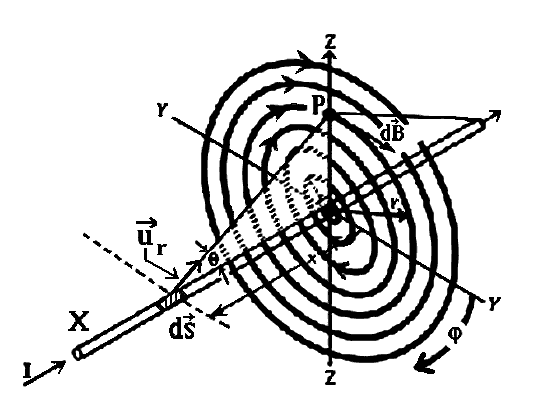Figure 1
On figure 1, the constant electron currentflows along the X-axis.  This electron current generates an element of magnetic field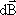(at location P) perpendicular to both, the electron current inand also the elementary vector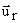joining the elementto P.  The magnetic lines of force surrounding the axis of the electron current in the plane Y-0-Z, are represented by heavy circles.  Also, passing at P, where the magnetic field is calculated, makes an angle q, with respect to the elementon the X-axis.  Angle  j is the angle around the X-axis.
---
In the Biot-Savart’s law, the magnetic fieldis always perpendicular to the X-axis.   The Biot-Savart (1) law is given by the relationship:1
Equation 1 gives the componentof the magnetic field (at P), at a distance r from the X-axis.  This magnetic field component is perpendicular to both, the elementand the elementary vector.  Therefore the intensity of the magnetic field in P, given by the Biot-Savart equation (eq. 1), is proportional to Sinq, because it takes into account only the component of the magnetic field perpendicular to the element of currentAfter the initial transient, when the flow of electron current is stabilized in the wire, no more energy is directly required to maintain that induced magnetic field.  That can be verified in the case of an electron current inside a conductor, since it is well known that the current (and therefore the magnetic field) remains naturally constant in time, if the electric resistivity of the conductor is zero, as in the case of a superconductor.  In the case of a conductor with a non-zero electrical resistivity, the energy required to maintain the current, is totally used to heat up the wire.  However, if the electron current is formed by a free electron beam, traveling in vacuum, it is even more obvious that the current of free electrons maintains a constant velocity inside the electron beam, due to momentum conservation.  Since the Biot-Savart equation 1 is most suitable to calculate the magnetic field, with any electron current, we can consider either a current generated by the flow of free electrons in vacuum (as for the electron beam drifting inside a cathode ray tube), or the constant electron current inside a wire.

2 - Magnetic Field Produced by Single Moving Electrons.
Using the Biot-Savart law, let us now calculate the magnetic fieldwhen we have an extremely small current.  We consider the special case of a magnetic field produced by an electron current formed by one single electron, moving at velocity v. Therefore, the long linear distribution of electric charges in the Biot-Savart equation must be substituted for one concentrated electric charge existing at one point.
We know that the electric current I, is defined as defined as a number of individual electrical charges (e-) passing through a point per second. Since the electric charge is quantized, in the case of a single electron it is impossible to calculate an infinitesimal variation of charge of one single electron.  Electrons cannot produce a continuous flow of electric charge.  This is particularly obvious when the number of electrons is close to unity.  In one unit of electron current, (one ampere) we have N(1 amp)@6.25 x 1018 electrons.  The electron current I, is defined as the passage of a charge Q coulombs of electric charge per second.  We have:2
Since the electron charge is quantized, the manifestation of one new individual electron corresponds to the appearance of a new charge dQ.  Therefore dQ=d(Ne-). The electron velocity v is defined as the distance dx traveled by the electric charge along the x-axis per second.  In the Biot-Savart equation, since the electron current I, is constant during one calculation, its velocity is also constant (v=constant).  We can write: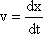3
Equations 2 and 3 give: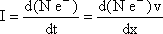4

The scalar form of the Biot-Savart equation is: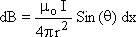5
Substituting equation 4 in 5 gives: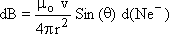6
Equation 6 shows that when we introduce a new linearly distributed electric charge d(Ne-), a new magnetic field dB appears at distance r in the direction q with respect to the unitary vector.  We have seen above that without the integration of the electric charges along the x-axis, the Biot-Savart equation, gives the increase of magnetic field dB produced at a distance r, due to the velocity of an elementary electric charge dQ distributed along length ds.  Since we calculate the magnetic field generated by one single (isotropic) electric charge, the linear distribution of charges used in Biot-Savart equation no longer exists.  Therefore equation 6 must be modified to take that into account that change of geometry of the electron source.
Quantization of Charges - Equation 6 gives the component of the magnetic field B in direction q, produced by one electric charge composed of N electrons distributed along the vector. In Maxwell’s time, it was unknown that the electric charges were quantized.  Since the electric charge is quantized in individual electrons, the fundamental assumption of a continuous distribution of charges alongassumed a century ago is incorrect. However, since there are about 1019 electrons in one unit of electron current, there is generally no appreciable difference, whether we consider that large number of individual charges or a continuous flow of charges.  Yet, in equation 6, we wish to consider a number of electrons as small as N=1.  Equation 6 must be re-considered in order to look for that necessary adjustment, due to the disappearance of the linear distribution of electric charges given by vectoras a consequence of the quantization of the electron charge.
It is interesting to note that H. Poincaré (2) in 1906, was the first to recognize that another force, called the “Poincaré stress” has to be present to prevent the electric charge of an electron from flying apart due to the Coulomb repulsion.
Since we now consider the magnetic field produced by one single quantized electron, additional transformations are also required.  For example, the Biot-Savart equation defines the magnetic fieldin a direction with respect to the element.  In the Biot-Savart equation, the electron charge distribution is not isotropic.  From the explanations above, in the Biot-Savart equation, we have seen that the Sin (q) function (Eq. 6) determines the direction of the calculated magnetic fieldwith respect to the continuous charge distribution.  However, when we have a single electron, it becomes impossible to define the direction of a no-longer-existent continuous distribution of electric charges. Since the axis of distribution of the electric charges no longer exists, we have to find the new geometry.   Since we now have an isotropic electric field around an individual electron, let us assume that the magnetic field generated is also isotropic. Eq. 6 becomes:7

Here, dBi is the magnetic field calculated considering quantized electric charges, but also the fact that the axis of distribution of the electric field no longer exists.  Equation 7 gives the total magnetic field dB for an isotropic geometry around a single free moving electron when the moving electric charges generating that field are also isotropic.
We can easily visualize qualitatively that the magnetic field produced by a row of electric charges is, as calculated by the Biot-Savart equation, not isotropic, because the magnetic field generated backward by the particles in the first part of the row of charged particles cancels out the magnetic field generated forward by the ones in the last part of that row of electric charges.  Of course, that cannot exist for a single electron.  It is not surprising that the passage from a geometry of linearly distributed electron charges (in an electron current) to the geometry of a point source (single moving electron) produces a similar change of geometry in the resulting magnetic field.  We will see below that the hypothesis above is valid because the isotropic distribution of the magnetic field around single electrons is compatible with equation 1, (which is applicable to a smooth linear distribution of electrons).
Experimentally, due to its smallness, it has never been possible to measure the faint magnetic field induced around one single moving electron.  Nevertheless, the validity of that relationship is verified indirectly by the correctness of the Biot-Savart equation. We remember that the Biot-Savart equation was planned only to calculate the component of the magnetic field appearing in a specific direction, in the case of a large number of electrons distributed linearly.  It was not destined to calculate the total magnetic field around one single isolated electron.  The existence of quantized electron charge in an electron was still unknown in Maxwell’s time.  Only the effect produced by a continuous flow of electric charges could be considered then.  Consequently, this calculation here, which involves independent electrons, is more fundamental than the Biot-Savart equation, since the fundamental corpuscular nature of the electric charge, which is more realistic in physics, is now taken into account.

3 - Induced Magnetic Energy around a Single Moving Electron.
We have seen that equation 7 gives the amplitude and the distribution of the total induced magnetic “field” around individual moving electrons.  Corrections have been made in order to take into account that we have now point like electric charges so that the electric charge of the electron is no longer distributed along a line.  Also the electric field around one electron is isotropic and is not distributed along a line as in the Biot-Savart problem.
These new considerations are such that the Biot-Savart equation is still valid when the electric charge is distributed as a continuous flow. From that induced magnetic field, let us calculate now, the magnetic “energy” around one single (N=1) electron.   The density of magnetic energy um which is defined as the magnetic energy Um per unit volume V, is given by the relationship (3)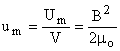8
Since the magnetic field d(Bi) around a single electron calculated in equation 7 is the only magnetic field of interest in the rest of this paper, we simplify the notation below substituting the magnetic field dB in equation 7 by the plain symbol B.   Around one single electron (N=1), from Eqs. 7 and 8 we calculate the magnetic energy dUm inside a volume dV.  This gives: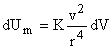10
with11
Equation 10 gives the magnetic energy dU in volume dV around one electron.

4 - Total Mass of Magnetic Energy in One Single Moving Electron.
Equation 10 gives the total energy of the induced magnetic field around one moving electron in volume dV.  Let us calculate the total mass M of that magnetic field surrounding the moving electron, using the Biot-Savart’s equation.  We know that the constant of proportionality between energy and mass is c2 (in the relationship E=mc2).  Since equation 10 gives the energy per unit volume dV, it must be divided by c2 in order to get the mass of the magnetic field.  We have: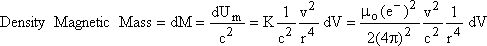12
Let us calculate the total magnetic energy (and mass) in all that infinite volume around a single electron.   Since the integration of equation 12 contains a singularity, we must find the appropriate integration limits of magnetic mass.  In electromagnetic theory, the magnetic field around a moving electron (Eq. 7) extends up to infinity. Therefore, the total mass of the magnetic field surrounding the electron must be integrated, in all three dimensional space, up to infinity.  We have seen above, that the distribution of magnetic energy is compatible with an isotropic distribution, since the electron has a spherical geometry. In order to integrate the total mass of the magnetic field of the moving electron, we use the integral of the volume of a sphere, on which we apply the variable radial density calculated in equation 12.
On figure 2, we see that the differential surface element of the surface of a sphere, at a distance r, is equal to a thin square, having sides respectively equal to r d along meridians, multiplied by the element of longitude j of the circle 2prSin(q).---
Figure 2
Figure 2 illustrates the parameters used in equation 13.
---
The total volume of an ordinary sphere is given by the double integral: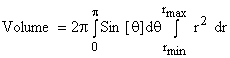13
In equation 13, the volume of a sphere between radius zero and radius rmax is, as expected: V=4pr3/3.  In the case of magnetic energy, which extends to infinity, the upper integration limit of the radii in equation 13 is infinity.  We must then take into account that the density of the magnetic mass is variable and decreases as 1/r4, as given in equation 12.  In order to calculate the total mass of electromagnetic energy, inside a volume given by equation 13, we have to integrate the mass density distribution in equation 12, with the volume in equation 13.  This double integral is integrated in the following way: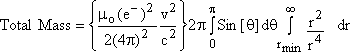14
Equation 14 can be written:15
The total mass of the magnetic energy in equation 15 remains finite even if the upper limit of integration is infinity.  However, we notice that equation 15 gives an infinite mass (magnetic energy) at r = 0.  We know that an infinite mass is not physically realistic. There is obviously a physical constraint, which must now be taken into account.
Magnetic fields are well measured experimentally at any large distances, but such a measurement is no longer possible directly at an infinitesimal distance, very near the center of the electron.  At r = 0, equation 15 shows that it would require an infinite amount of energy to generate magnetic fields right down to the center of the electron.  Therefore, the total electron energy of 511 keV, gives the information of how close to the geometrical center, the field can exist.  As a result, a hollow structure of electric and magnetic fields of the electron is absolutely necessary, due to the finite energy of the electron (511 keV).  More explanation is given below in section 7.  There is a minimum radius expected due to the fact that the electron energy is finite (511 keV), which is called the classical electron radius (re) (3).   There cannot exist any electromagnetic energy within the classical electron radius (re), because the velocity of that empty part of the electron (hollow cavity) cannot induce a magnetic field as calculated by the Biot-Savart equation.
Let us integrate the magnetic energy from the well-known (hollow) classical electron radius re up to infinity, where an electric field can exist.  From equation 15, we have: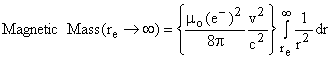16
After integration, this gives:17
Equation 17 gives the total mass of the magnetic field in a single electron, moving at velocity v.

5 - The “Relativistic Increase of Mass”.
We wish to compare the magnetic mass of a moving electron given in equation 17, with the kinetic mass (which is the increase of the so-called “relativistic mass”) of the same electron.  When we apply the principle of mass-energy conservation, we have found (4), that the mass of a moving particle in motion Mv is given by the same relationship as in Einstein’s relativity.  The mass of a moving particle is given by the relationship18
Where g is: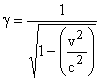19
From equation 18, the increase of mass due to velocity, is: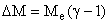20
In mathematics, we can show that a series expansion of equation 19 gives:21
Equations 18, 19, 20 and 21 give: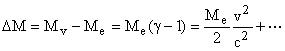22
In equation 21, the second order term (v/c)4 is extremely small when v is much smaller than the speed of light.  The (v/c)4 and others higher order terms, are negligible with respect with the first term.   It can be shown that these higher order terms are due to the energy required to accelerate the increase of mass due to the previous order term (v/c)2. For the moment, let us neglect the (v/c)4 and higher order terms.

6 - The Magnetic Mass Versus the Relativistic Mass.
Let us compare the increase of magnetic mass calculated above in equation 17, with the increase of electron mass, using relativity theory as given in equation 22.  In fact, we are testing whether the relativistic mass is the same thing as the magnetic mass.  Equations 17 and 22 give:23
We notice in equation 23 that both phenomena (magnetic energy and relativistic energy) produce an increase of mass, which is proportional to (v/c)2.  This means that the magnetic energy around individual electrons increases as the square of the electron velocity, just as the increase of relativistic mass.  To be totally identical, it remains that we need then to compare “only” the constant of proportionality between these two phenomena.  Simplifying equation 23 gives: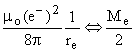24
One quick way to verify the equality in equation 24 is to use numerical values.  The best accuracy known data are: The permittivity of space is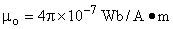. The electron charge is: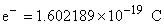.  Finally the classical radius of the electron, given in tables is:Let us substitute these constants and the electron mass, which is Me= 9.109534 x 10-31 Kg. This gives: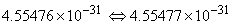25
Equation 25 shows that the magnetic mass of the moving electron is, within experimental accuracy, identical to the increase of relativistic mass for any velocity of the particle.  Such a striking agreement cannot be a coincidence.  We conclude that the two quantities in equation 23 are physically identical.  Those two quantities are identical at any velocity of the electron as explained above.  In both cases, there is an identical increase of mass with respect to the electron mass at rest.
Therefore the increase of the so-called relativistic mass is in fact nothing more that the mass of the magnetic field generated due to the electron velocity.
In fact, the real fundamental nature of the kinetic mass, which increases with velocity, is nothing else than the magnetic energy, as given by the Biot-Savart equation.  From equations 17 and 23, we can conclude that for an electron, the physical nature of the parameter g in relativity is:26
The so-called “relativistic increase of mass” is only due to the induced magnetic field as calculated by the Biot-Savart equation.

7 - Physical Meaning of the Classical Electron Radius.
Let us examine a natural interpretation to the Classical Electron Radius.  Following the above calculation (re in eq. 17), we see that the “Classical Electron Radius” can be described, as the size of a central cavity with radius re, in which there is no field, because this would require an amount of energy (and mass) which is not compatible with the electron mass.  An infinite amount of energy is required if we assume that there is a field at the center of the electron, inside that cavity.  This is physically unrealistic and therefore impossible.  The fact that there is always only 511 keV of energy available in an electron is a natural physical constraint, which prevents any electrical field inside that classical radius.
It has been shown that electrons at rest are pure electromagnetic fields  (3).  Since the electron is pure electromagnetic energy, the total electromagnetic field surrounding the electron has an energy equal to
U=mec2=511 keV.  Using that relationship (3), the entire energy of an electron is:27
Then the electric field can be accumulated by integration, bringing together these infinitesimal electromagnetic elements forming the electron.  We find that the size of the cavity decreases when the charge gets larger.  Due to Coulomb repulsion of electric charges, energy is required to bring together the elements of electric field forming an electron. Since we know that the total energy of a single electron is 511 keV, the total energy required to compress all the electromagnetic field toward a concentrated packet (which is the electron) is limited to the total energy available to an electron.  It has been calculated that when the total energy of the fields, reaches the electron energy of 511 keV, the electric charge of the electron is then complete, so that no further electric field can be accumulated in the inner region of the electron. Therefore, the charge distribution forming an electron has the shape of a hollow sphere, having a radius ro(e-), called “classical radius of the electron” (3).  Outside the “classical radius of the electron”, the electric field (of an electron at rest) decreases smoothly (as 1/r2) to zero at an infinite distance, as observed experimentally. The fact that the electromagnetic field is absent inside the classical radius is demonstrated in the high-energy electron scattering experiments (much above 511 keV), which show that the electrical potential of the electron does not reach an infinite limit (at r=0), but remains below 511 keV.  The interaction between particles is due to the interaction between the fields located at the exterior of the classical radius.  That way, there is no need of existence of a paranormal action-at-a-distance hypothesis.  At a distance, it is the exterior parts of the particles, which are always reciprocally interacting.

One must logically conclude that the electron is made of fields surrounding a hollow core.  The entire electron field is exterior to that radius.  When we simulate the formation of an electron from a gradual integration of an electromagnetic field (3), we notice that the intensity of electric field outside the central cavity must always remains constant, independently of the total electromagnetic mass accumulated in the particle.  This explains the fact the electric charge always appears with a constant amplitude for all particles (electrons or protons).  It is the size (radius) of the empty cavity, which decreases when new electric fields are integrated.  In agreement with physical data, outside the central cavity, the electric field around the electron is identical (in amplitude) to the electrical field around the proton.
We normally have the impression that the amount of integrated electrical charge in the electron is identical to the amount of electric charge in the proton. However, it is just the external part of the electrical field (larger that the classical electron radius), which has the same amplitude.    The proton possesses a much larger amount of charge (mostly responsible for the larger proton mass) located in the gap between the classical electron radius and the classical proton radius.  The addition of some extra electric charge to the electron, at a location inside the classical electron radius, to form a proton (or anti proton) does not change the amplitude of the remote electric fields around the newly formed particle.
Consequently, we must realize that the physical concept of “charge” must be reconsidered.  It must not be confused with the remote electric field, which is always the same around any electric charge. We usually claim that the electric charge of the proton is equal to the electric charge of the electron, just because the field distribution is identical at r>re. We have seen that when more electromagnetic field energy is integrated inside the proton, within the classical electron radius re, there is no change of remote electric field around the particle.  Furthermore, we must understand that the electric field around a charge particle is not due to the “action-at-a-distance” around an electric charge located at one point.  An “action-at-a-distance“ implies an interaction between two physical elements located at different locations.  That is non-sense. Logically, the interaction between two elements can take place only, if they are located at the same place, at the same time.  The electric field, which is located around a charged particle, possesses its own existence at the location where it is measured.  It is not some magic “action-at-a-distance”.   An interaction between “particles” can take place only when the field of an interacting particle has reached the inside of the interacted particle.  It is always a field-to-field interaction at any distance.  Such a mechanism solves the “action-at-a-distance" paradox.
In Jackson (3), when the mass of the field is equal to the observed mass of the particle to the extent of the charge distribution, we find that the minimum radius is the classical electron radius ro(e-), where: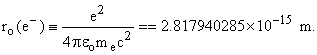28
The description above shows that the entire mass of the electron “at rest” is a distribution of electromagnetic field, surrounding a hollow core.
This is illustrated on figure 3.As explained above in section 6, we can add that the relativistic mass-increase, due to the mass of the magnetic field, is in agreement with the Biot-Savart equation.  We must also conclude that there exists no massive nucleus at r=0 inside the electron.
---
Figure 3.
Figure 3 illustrates the cross section of an electron at rest, representing the electric field density by a dark area.  The electric field of an electron at rest is isotropic (in 3-D) around its hollow core and extends to infinity.
---
Let us compare the above model, which is deduced from experimental observations and mass-energy conservation, with some previous theoretical electron models.  There have been many theoretical attempts to discover the internal structure of electrons.  For example, Poincaré(2) was the first to recognize that another force, (called the Poincaré stress) has to be present to prevent the electron from flying apart due to the Coulomb interaction.  The same problem has been studied by M. Abraham (5).  Of course, in principle, the magnetic component of the field might produce stability, but we still do not know exactly how.
More recently, different electron models have been suggested (6-12). All these mathematical models suggest that the electron is formed with electromagnetic fields.  However, none of these models can give a coherent description of the force capable of holding together the electric charge (the Poincaré stress).  The field forming a hollow electric sphere is formed with a self-repulsion electric field, but the fundamental origin of that Poincaré stress(2) is still missing.  Even in the most recent paper by J. G. Williamson, and M. B. van der Mark(11) , they attempt to give the electron charge distribution in the nucleus.  The toroidal geometry proposed for an electron at rest does not lead to the accurately observed isotropic decrease of electric field around an electron.  Furthermore, this model does not seem compatible with some observations and with the de Broglie phenomena discussed below.  Whatever is the nature of the Poincaré(2) stress, which holds the electric field together, we know with certainty that something holds the elastic electric field together because it is an observed experimental fact that most of the electric charge of electron is concentrated inside a localized volume of space.   Finally, experiments also show that the total amount of electric charge in particles is the same for electrons, positrons, protons and anti-protons.  Therefore the above model (fig. 3) is compatible with experimental data, which shows that both are in agreement with a quadratic decrease of the electric field around the electron, and also with the principle of conservation of mass-energy, which requires that the total energy of the electric field of the electron, is compatible with the electron mass.
In order to reach a deeper understanding, the electric charge distribution forming the electron must be compared with another particle.  A similar electric field distribution takes place in the proton and the anti-proton, which also possess an electric charge.  Even for the proton, the (positive) electric field cannot exist down to zero radius, because this would also require an infinite amount of energy.  However, at a larger distance from its center, up to infinity, the proton electric field possesses exactly the same amplitude as the positron and the electron.
Since the proton mass is much larger than the electron mass, it takes the integration of a much larger amount of electric field to form the proton mass.  However, we know experimentally, that in the outer region of these two particles, the density of the electrical field is exactly identical.  Therefore the proton possesses an extra electric field, entirely located just inside the classical electron radius, which gives it the extra mass.  That extra inner charge does not produce any effect on the peripheral part of the particle.  At distances larger than the classical electron radius, the “amplitude” of the electric field surrounding the proton is exactly the same as for the electron.  This is in agreement with the observations that the (absolute) electric charge is the same for both particles.  Except for the polarity of the electric charge, the important difference between the electrical fields making up an electron and a proton, is that the central cavity inside the electric fields is much smaller for the proton than for the electron.  In the case of the proton, the mass of the electric field is accumulated until the proton mass is reached.  However, the surrounding electric field is the same for both particles, which is interpreted as having the same charge.  In fact, only the amplitude of the surrounding “field” are the same.
This consequence is in agreement with high-energy scattering experiments.  Protons can show a much higher electrical potential (about 1000 MeV), than the electron (0.511 MeV), when they are scattered at very high energy.
An illustration of the proton at rest is similar to figure 3, except that the cavity inside the particle is much smaller.   In the same way, as in the case of the electron, the classical radius of the proton is: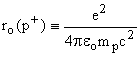29
The numerical classical proton radius is:
ro (p+) = 1.53470 x 10-18 m.           30

8 – Vortices in the Electric Field of Moving Electrons.
We understand from the above, that electrons, positrons and protons are not point particles, but kinds of hollow clouds of electric fields.  In order to accelerate an electron, we need to have an interaction with the mass, which causes the acceleration.  Let us consider a simple model of interaction when the electron is accelerated.  When the central part of the electron (around ro), where most of the energy is concentrated, interacts with another particle, this is somewhat similar with the interaction of a body (a stone) falling into a water pool.  In physics, we know that all the momentum as well as the kinetic energy of the projectile must be transferred to the water pool.  For the sake of simplicity, we mention a falling stone, but a better analogy would require a penetrable jellylike object.  In the case, in which the internal motion (vortices) inside the fundamental particles is produced, we do not observe that the kinetic energy is immediately transformed into heat, contrary to the case of putty projected on a hard surface.  For example, the waves in water do not appear as thermal energy as long as they remain waves.  When the falling stone reaches the surface of the fluid, the increase of pressure under the stone pushes water away from under the stone. The pressure in the fluid pushes water in a radial displacement, and water is forced out horizontally from under the falling stone.  That water, from under the stone, provokes a horizontal radial motion forming a wave ring of fluid.  These doughnut-shape rings consist in a whirling fluid rotating around circular axes, which are called “toroidal vortices”.  As soon as the falling stone moves deeper through water, all the way to the bottom, many more vortices are formed inside the fluid.  The formation of these waves at the surface, as well as in depth, must satisfy the energy and momentum conservation.
Circular waves can be easily seen at the surface, as a function of time, as illustrated on Figure 4.  In this paper, the vortices are illustrated qualitatively.  Mathematical calculation of vortices is already known mathematically.---
Figure 4
Each circle represents one of the vortices at the same location, at different times.  The black dot on each circle represents an oscillating drop of fluid rotating around the vortex.
---
Figure 4 is a dynamic illustration of the toroidal vortices, well known in hydrodynamics.  This is similar to the problem of waves traveling at the surface of water.  Those vortices are visible at the surface of water, but other similar vortices are also produced at all depths.  At the surface of water, we have the illusion that the waves move away from the center.  However, hydrodynamics has shown that it is an illusion. In that case, the center of the wave-system would then become depleted of water, which does not happen.  Figure 4, illustrates two full rotations of a drop of fluid inside a vortex, during a time interval corresponding to the passage of two waves.  Therefore the kinetic energy of the stone falling in water is transformed into the kinetic rotation of the fluid inside these rotating vortices.  This is the way energy is conserved.  In water, due to the low coefficient of viscosity of the fluid, the kinetic energy induced in the fluid is not readily transformed into heat.  In order to be compatible with the principle of energy and momentum conservation, in the case of high fluidity, there exists no other physical mechanism, than forming those toroidal vortices.  That is the way the kinetic energy of the stone is transformed into the kinetic energy of the fluid, in vortices.
A similar phenomenon also exists in other fluids as in air, which also has a low viscosity.  In that case, the kinetic energy of the wind is transformed into vortices called whirlwind, twisters, and tornados, well before the energy is finally transformed into heat.   However, if we chose a fluid with zero viscosity (like low temperature superfluid liquid helium), all the kinetic energy and momentum of the falling mass remains permanently under the form of vortices in the fluid.  In the case of superfluidity, the motion in the fluid is never transformed into heat.  Due to superfluidity inside electric fluids, there exists no mechanism, which can transform the vortices of electric fields into heat.  Thermal energy does not exist at the nuclear level, inside elementary particles.  Therefore, the electric field forming an electron must also be a superfluid.  With zero viscosity of the electric field forming the electron, kinetic energy inside vortices can be conserved indefinitely, due to these superfluid toroidal vortices inside each electron.   Without storing the kinetic energy in those vortices, it is impossible to satisfy the principle of energy and momentum conservation.
We have seen in equation 17, that when an electron is accelerated, that moving electron possesses kinetic energy, which is equal to the magnetic field induced by the electron, and which is also equal to the relativistic mass.  Now, from the above considerations, we see that when the electron is accelerated, some vortices must necessarily be formed in order to carry the kinetic energy given to the particle.A more general overview of these oscillations, showing the toroidal vortices, is presented on figure 5.
We see several toroidal vortices created by the absorbed kinetic energy given to the electric field inside a non-viscous fluid.  As demonstrated above, these vortices possess the kinetic energy, which appears as magnetic field, and which corresponds to the relativistic mass.
---
Figure 5.
Figure 5 illustrates a few internal toroidal vortices inside a moving electron.  The kinetic energy absorbed during the acceleration of that electron becomes the energy of the toroidal vortices, which is also the magnetic energy calculated with the Biot-Savart equation. With decreasing amplitude as a function of r, these electric and magnetic fields extend up to infinity.  The energy of these toroidal vortices (the magnetic field) is equal to the relativistic mass as demonstrated above.
---
Figure 6 illustrates the internal structure of the first three vortices drawn on figure 5.  Figure 6 illustrates the vortices, assuming a perfect conservation of energy and momentum, when the electron has been accelerated by a force “F” applied (downward) on the central part of the electric field of the electron.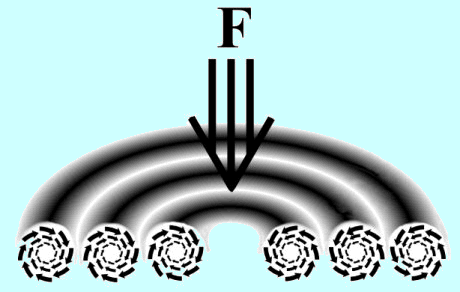---
Figure 6
Figure 6 illustrates an electron after acceleration by an external force F giving velocity v, on the axis of the electron current. Figure 6 shows the first vortices of an electron.  All the other concentric vortices decreasing as 1/r (for an electric current as in Biot-Savart equation), are not drawn.
---
Applying the principle of momentum conservation, we observe, on figure 6, clockwise vortices of the electric field on the left hand side of the figure, and anticlockwise motion on the right hand side.  It can be shown that this is also compatible with the fact that a magnetic field has an opposite direction, on the opposite side of a flow of electric charges generating a magnetic field.  We can see that the fundamental nature of a magnetic field is nothing else but an internal velocity of the electric field, forming vortices at large distances as shown by the Biot-Savart equation.  Again, due to the zero viscosity of the electric fluid, the vortices inside the electron field are permanent internal rotating electric vortices forming waves, which store up the kinetic energy induced by the interacting particle.  We will see below in section 10 that these vortices are also in perfect agreement with the well-known de Broglie wavelengths.

9 – Absolute Frame of Reference without Ether.
In section 8 above, we have seen that, when an electron is accelerated from rest to velocity v, the principle of energy and momentum conservation requires that vortices of electric fields be created inside each charge.  As explained in section 8, these vortices are tensors, which involve rotations related to the direction in which the electron has been accelerated.  If a moving electron receives a further acceleration in the same direction, the number of vortices will have a further increase.  Also, if that moving electron moves sideways, the direction and the amplitude of the vortices rearrange accordingly, in order to always satisfy energy and momentum conservation.  Finally, if that electron is accelerated backward (slowed down), down to its initial zero velocity, these internal vortices of electric fields cancel out and disappear completely.  Therefore, we see that moving charges, always keep inside the particle, all the information about their speed and their direction as a result of electric vortices.  The full information about their speed and direction exists in the electric charge at any instant, and remains permanently inside all moving individual charges.  In fact, electric vortices are more than perfect gyroscopes, since they do not only record perfectly their direction of motion, but furthermore they record their velocity with respect to an absolute rest frame, because the energy in the vortices is an exact measure of their absolute velocity.  Therefore, “all” particles (electrons, protons atoms and molecules) possess individually and internally all the information about their speed and their direction, with respect to an absolute frame of reference.  This is the only way to assure the conservation of energy and momentum and compatibility with the induced magnetic field following the Biot-Savart equation.  Since the electric charges possess all the information about the absolute velocity, one must conclude that there exists an absolute frame of reference corresponding to zero energy of the vortices.  Without an absolute frame, it has been seen (4) previously, that there cannot exist any physical reality, which could be independent of the observer.  For example, in the Biot-Savart equation, with respect to what does the velocity “v” means?  Since a magnetic field is not an illusion and possess its own existence, it cannot possess a different energy as a function of the velocity of the observer. That would be incoherent, and therefore totally illogical.
We can read that in many papers, such a rest frame is attributed to a hypothetical “ether”.  However, there is a difference between an absolute frame of reference, which possesses only geometrical properties and a physical “ether”.  A “ether” must be understood as a “medium” which can have a physical interaction with other physical quantities, as mass and energy.  Ether is usually assumed to be a support for the transmission of light waves, in analogy with the transmission of sound, which is supported by air (or by solids and liquids).  However, acoustic theory shows that no sound can be transmitted through a medium, if that medium does not possesses mass.  Therefore some energy and some physical properties (other than geometrical properties) must exist in such a hypothesized ether, which can interact with the assumed waves moving through that ether.  To be realistic, ether, if it exists, must possess mass.  Therefore, there is a fundamental difference, between an “absolute frame of reference”, which is just a geometrical property, and which corresponds to the average motion of matter in space, and a “physical medium”, possessing physical properties like mass and energy, which supports waves.  It has been shown previously (15) that an absolute frame of reference (not the ether) is required in order to satisfy the principle of mass-energy conservation.  We read:
“ For the moment, the sole property of that assumed ether is to establish an absolute origin to the velocity-frame of light and physical matter, because this frame of reference is absolutely needed to comply with the principle of energy and momentum conservation.”
However, as mentioned above, this is the “sole” property, which is needed, because the principle of mass-energy conservation is fully satisfied without any energy belonging to that assumed medium.  Then again, it is a geometrical property. Other phenomena have also been observed, which shows that an absolute frame of reference is required without the interaction of any physical medium (ether).  The GPS (16), which requires a non-relativist correction (because it requires the Sagnac effect), provides a proof of a need of an “absolute frame of reference” for light propagation without involving any interaction with the media.  It is shown that the velocity of light is actually (c-v) in a frame moving at velocity v, (17, 18) even if the moving observer always measures c in his own frame.  In fact, the velocity of light is an absolute constant in an “absolute frame” at rest, but due to the different clock rate in the moving frame, there is an apparent velocity of light equal to c in all frames (16).
It is found that although an absolute frame of reference is required to be compatible with the principle of mass-energy conservation, there exists no proof that an ether, which would fill vacuum, exists.   Of course, some undiscovered extremely weakly interacting fields or particles could exist in space.  However, since the principle of mass-energy is well satisfied in current experiments, these unknown fields do not appear to be responsible for the transmission of light and particles in vacuum.

10 – Coherence between Vortices and the de Broglie Wavelength.
We have seen on figure 5 that the vortices developed inside moving electrons generate naturally a permanent wave structure inside electrons moving at constant velocity, exactly like the wave structure required by the de Broglie equations.  Therefore, the internal wave structure of a moving electron can be interpreted as matter-wave, as predicted by the de Broglie wavelength equation (13), and well observed experimentally.  Just as in the case of a stone falling into a water pool, the wave structure of the electron vortices, which is also compatible with the magnetic field calculated using the Biot-Savart equation, is the only way to take into account simultaneously the principle of energy and momentum conservation inside the electron.  Since there is no inelastic mechanism inside an electron to give up the kinetic energy of these vortices, these vortices remain permanent as long as the inertial velocity of the electron is maintained.  The electric field distribution of an electron “at rest” is illustrated on figure 3, which corresponds exactly with an infinite wavelength, as predicted theoretically by de Broglie.
We must also note on figure 5, how an electron becomes compatible with electron diffraction, which requires that the electron possesses a large oscillating transverse structure as well as a wavelength in the axial direction, which are velocity dependent, as observed experimentally, in agreement with the de Broglie equation.    Consequently, we can say that the de Broglie wavelength of an electron is due to the magnetic component, which exists under the form of electric vortices of moving electrons.  This mechanism, generating vortices so frequently observed in water waves, possesses many similarities with the toroidal vortices of the electric field inside each moving electron.  Most importantly, we must take into account that the electric fluid is a super fluid.  The de Broglie electron wavelength as a function of velocity is given by the relationship: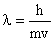31
Using equation 31, we see that we can calculate the density of the de Broglie wavelengths, per unit length.  Just as for toroidal vortices, equation 31 shows that the number of de Broglie wavelengths per unit length is proportional to the velocity of the particle.  Therefore there is a striking agreement that the induced vortices in the electric field of the electron are responsible for the de Broglie wavelengths of particles.
We have seen that due to the principle of energy and momentum conservation, the number and the intensity of vortices increase with electron velocity.  Therefore, when the electron is accelerated to velocity v, it is observed that that electron becomes different, because it acquires vortices, which give a proper wavelength to the electron.  As seen above, these vortices are also the magnetic field generated by a moving charge according to the Biot-Savart equation.  Therefore, the de Broglie electron wavelength is the wavelength of the vortices, which is responsible for the density of the vortices, and therefore the magnetic field.
The wavelength of these vortices in electrons is what produces the electron diffraction in matter.  This is shown in atomic and molecular physics, since the length of the electron’s orbits inside the atom is an integer number of the de Broglie electron wavelength.  The de Broglie relationship has been first used in the Bohr model to establish the basis of quantum mechanics.  In the Bohr atom, it is well established that the length of an electron orbit of a particular quantum state, is always equal to an integer number of de Broglie wavelengths.  The de Broglie wavelength is equal in size to the wavelength of the vortices (see figure 5), inside the moving electron.
It can also be seen that the internal electric vortices of electrons have a similar structure as the electron spin.  These spins can be coupled with other interacting spins forming quantum states. The wave nature of the vortices inside electric charges is also observed in atomic, molecular physics and nuclear physics. The vortices forming the spin of the proton are also coupled with the electron vortices (spin) in the hydrogen atom, to form the 1S and 3S states (of the ground state), depending in the relative orientation of the vortices (spins).  It is not surprising that the energy states of atoms and nuclei are quantized, since we can see in mathematics that the switching from one configuration of vortices to another one is discontinuous.  Therefore, each coupling between different pair of vortices (of neighboring particles), which requires a different amount of energy, is the fundamental explanation for the quantization of energy.  This is in perfect agreement with quantum mechanics.

11 – Application:  Physical Model of the Photon.
Relativistic Mass. - We have demonstrated here that the magnetic energy generated by the velocity of electrons, possess a mass, which is identical to the increase of the so-called relativistic mass in Einstein's relativity. Also, as demonstrated above, the energy of the magnetic field is the energy of the vortices of the electric field.   Therefore, the fundamental phenomenon responsible for the increase of relativistic mass with velocity is the magnetic vortices of the electric field, as calculated with the Biot-Savart equation. Consequently, since the magnetic field can be observed at distances of many meters around wires carrying the current, in agreement with the Biot-Savart equation, the mass of each electron is equally distributed in a corresponding huge volume of space.
Super Fluidity of Electric Field. - We have seen that the electric field forming an electron is like a drop of a superfluid, (like liquid helium), which has zero viscosity.  Considering the sub-atomic scale, there exists no physical mechanism, which can transform that tumultuous motion (vortices) inside the electron into heat.  The electric fluid forming an electron is a superfluid, with a decreasing density around the central radius.  However, we have also shown above, that almost all the electron mass is located in an extremely small volume.  For example, in section 4 above, we find that 99.99% of electron energy appears within a radius of 2.8 x 10-11 meter.  No doubt, it is this extreme smallness in the distribution of the energy of that particle, which is responsible for the belief that it might be a point particle.  An electron is nothing but its electromagnetic field.
However, considering the infinite distribution of the electric field of an electron in space (density decreasing as 1/r2), we must realize that each electron extends out to infinity.  Because of its huge size, the electron can interact, although sometimes imperceptibly, over an extremely large cross section.  This is in agreement with the vortices of electric field (called "magnetic field") measured many meters away from the location, where the electric flow is moving, as calculated using the Biot-Savart equation.  Due to the finite velocity of transmission of electric forces, the mechanism of acceleration of the electron must be described as taking place first, as the acceleration of the densest part of the field, where almost all the energy is located, before that acceleration is transmitted gradually toward the remote, much less dense parts of the particle.  Therefore electrons, as well as any charged particle, are not point particles.  On the contrary, the size of all particles extends up to an unlimited volume of space, since the electric field decreases as 1/r2.  Finally, that electric superfluid is held together by a force called the “Poincaré stress” (2), which keeps the electric charge from expanding away, and maintains the decrease of electric field according to the well-known quadratic law, up to infinity.
Internal Structure of the Electron. - It is well known experimentally that the static electric field around an electron “at rest”, decreases as 1/r2.  In physics, electrons are believed to be pure electromagnetic field.  Unfortunately, it is taken for granted, that the field around electrons “in motion” also decreases smoothly as 1/r2.  We can see that this is a hypothesis, which is not compatible with observations and with the vortices required for the application of the principle of energy conservation explained above.  Of course, measuring the distribution of the electric field around a fast moving electron can be extremely challenging.  However, many experiments have shown that moving electrons possess a structure compatible with the vortices explained above.  How can physicists assume that the electron always possess a smooth (according to 1/r2) structure, while the de Broglie equation shows that there is an internal wavelength, which inescapably belongs to the moving particle, as seen in electron diffraction experiments?  Furthermore, the wave structure of the electron is an experimental fact also observed inside atoms.  For example, when the electron inside the hydrogen atom is moving at velocity v around a nucleus, the de Broglie electron wavelength is equal to an integer number of wavelength, as required in the Bohr model.  This means that, “only” at some particular electron velocities, the electron vortices produce stable electron configurations in atoms.  At all other distances from the nucleus, the electron orbit is unstable.  It is contradictory to claim that an electron field decreases smoothly as 1/r2, while we know that moving electrons possess a variable wave structure, compatible with the de Broglie equation.  It is obvious that something inside the internal structure of the electron is changing as a function of electron velocity.  Since this is an experimental fact, supported by mass and energy conservation, it is necessary to consider that the internal structure of the electron varies with velocity.
A Realistic Model of the Photon.  - The fundamental nature of the photon can be understood using a mechanical description of the photon, on which we apply the laws of physics.  It is well known that electromagnetic radiation is always generated during the acceleration “a” of electric charges.  Using the Larmor equation, the energy emitted by an accelerated charge is given by the relationship: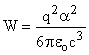32
Where q is the electric charge, and W is the power emitted in Watts.
We must avoid any confusion between the electromagnetic radiation emitted during the “acceleration” of electric charges (equation 32) and the Biot-Savart field (vortices) accompanying a moving charge at “constant velocity” (eq. 1).  During the acceleration, the electric charge can be completely free or bound to another particle. The emission of electromagnetic radiation from free accelerated electric charges is called bremsstrahlung.  Also, when the electromagnetic radiation is emitted from an accelerated electron bound to other particle, a corresponding emission of radiation also takes place. In that case, this is described as a quantum emission of radiation due to the transition between two quantum states.  Whatever the degree of freedom of the electric charge is, the emission of electromagnetic radiation always requires the acceleration of an electric charge.
The electromagnetic radiation emitted due to the acceleration of electric charges, bears many names.  It can be called: photon, light, electromagnetic radiation, cosmic rays, microwaves, radio waves, infrared radiation, ultra-violet radiation, etc.  All these different names refer to the same thing.  The difference in names is generally related only to the frequency of the radiation.  As mentioned in the book: “Absurdities in Modern Physics: A Solution” (14), it does not make sense to claim a physical difference between a photon, observed with a photon detector, (then considered as a particle,) and electromagnetic radiation, observed with a radiation detector, (then considered as a wave).  This always refers to the very same package of energy.  Under all these different names, there exists only one single fundamental phenomenon.  The “package of energy” emitted during the acceleration of a charge, must always be simultaneously compatible with a realistic description, corresponding to an electromagnetic field emitted by an accelerated charge, possessing all the characteristics of energy, amplitude, frequency, phase, length of coherence, time of coherence and polarization.  Therefore here, we use any of these terms indifferently, to represent electromagnetic radiation.  It is illogical to believe that the nature of light changes as a function of the detector or observer, as claimed unfortunately in many papers.
Since light (or photons or electromagnetic radiation, etc.) is always a consequence of the acceleration of an electric charge, the structure of light must be compatible with the morphological structure of the emitter, which is generally the electron.  In view of the fact that “moving” electrons are made of a large number of extended, concentric vortices of electric fields, this fact must be reflected on the morphology of the emitted radiation.  Each infinitesimal element of the accelerated electron emits energy in agreement with equation 32.  Due to the acceleration of a tri-dimensional volume of electric charges concentrated in vortices, as explained above, the acceleration of each differential element of the electron structure, gives rise to a tri-dimensional electromagnetic wave.  Therefore the structure of the emitted light (as wave-packets) must also possess concentric electric waves.  Since every parts of the three-dimensional electron are accelerated, similarly, the radiation emitted occupies all space, up to infinity, in order to be compatible with equation 32.  That is simple logic. Consequently, the so-called “photon” can neither be described as a point particle nor as an expanding field distribution around the source.  The so-called “photon” can be described as a non-expanding display of numerous concentric vortices (of unlimited radius) of electromagnetic fields in a three dimensional space, moving along the velocity axis.  Those arrays can be compared to the vortices produced, at different depths, when a stone falls into a water pool, as illustrated above.  However, this comparison is incomplete, unless in addition, we recall that it is the water pool, (with the vortices), which is moving at velocity v.  Then, the electric vortices of the accelerated electron generate corresponding vortices in the photon, which then move at velocity c. The velocity c of the electromagnetic radiation adds cylindrical parameters to the wave packet.  One must also consider the ends of this cylinder formed by the moving sphere, which also contains vortices, in compatibility with the morphology of the accelerated electron generating the “photon”.  We can see that the main length of the moving wave packet is the length of coherence of the radiation.  That length of coherence is related to the time during which the electric charge is accelerated.
Of course, the radius of the cylindrical vortices cannot expand in time, since this would decrease the density of energy in the photon’s field.  Experimentally, it is confirmed that the density of energy of a wave packet does not decrease in time, since it is an experimental fact that the quantum levels of target atoms are just as much excited at any distance from the light source.
We have seen that, just as in the case of the moving electron, a concentration of electromagnetic energy is also replicated in the wave-packet, at the instant it is generated by the electron.  Therefore, most of the energy in the wave-packet is concentrated inside a relatively small radius (just around the classical electron radius), but some energy also exists at large distances, up to infinity.  The fact that an individual wave-packet described here occupies a large volume of space (in both longitudinal and transverse directions) is such that it can interfere with itself after it has been split by a local barrier, like a slit.  Also, considering the very large size of the emitting electron, we can see that due to the finite velocity of transmission of energy at velocity c, the total energy of a wave-packet cannot be measured instantaneously, in agreement with the “uncertainly principle” in quantum mechanics.  Let us examine the fact that the so-called “photon” extends in the longitudinal, as well as in the transverse direction.
Michelson’s Interferometer.  - The Michelson interferometer is considered here to demonstrate the longitudinal wave structure of light. The Michelson interferometer produces interference fringes, by splitting a beam of monochromatic light with a half-silvered mirror placed at 45 degrees.  Therefore, light is sent off in two perpendicular directions.  One beam strikes a fixed mirror and the other a movable mirror.  Each sub-beam reflects off of another mirror, which returns it to the half-silvered mirror, where the two sub-beams recombine.  At the location where the reflected beams are brought back together, an interference pattern results.  When we displace the movable mirror, one axial section of the delayed light beam is superimposed to the other section of the same beam.  By moving the mirror, the delayed electromagnetic wave of a different section of the same wave-train, interfering with the wave reflected by the fixed mirror, produces the well known moving interference fringes.  In the Michelson’s interferometer experiment, most people do not realize that that experiment generally uses an atypical source of light.  If light from the movable mirror is delayed along a distance larger that the length of coherence, there exists no interference fringes.
This proves that each wave-packet of light contains an axial wave structure, of variable length, which is compatible with the model explained here.  It is also important to recall that these interference fringes exist at any light intensity, even if light intensity is as low as one photon per minute or per hour.  This has been shown experimentally.  Consequently, these interference patterns cannot be due to the interference between independent wave–packets. We must conclude that due to the longitudinal size of the light-wave emitted by a single electron, we can see how one single wave-packet of light, sometimes called “photon”, interferes with its own field, in the longitudinal axis.
Two-Slit Experiment.  -  It is also important to recall that the same wave-packet must also contain simultaneously a transverse electromagnetic field, in order to be compatible with the morphology of the moving electron, generating the wave packets. Consequently, we must show that experimental observations are also compatible with the interference between two different sections of electromagnetic field issued from the same photon, in which the transverse electromagnetic field also varies as a sine function in space.  This transverse electromagnetic field of the wave-packet can be observed experimentally with the two-slit experiment (or many-slits experiments).  In the two-slit experiment, we see that a different section of the wavefront of the same wave-packet must go across different openings on the two-slit-system, which is perpendicular to the velocity vector of the wave-packet.  Due to the transverse size of the wave-packet emitted by a single electron, some vortices of the same wave-packet interfere with others, having a longer or shorter path after passing through the slits.
This interference between the transverse fields inside a single wave-packet is well observed experimentally.  Of course, when the distance between the interfering slits is large, the possibility to produce interference gets much smaller in agreement with observations.  However, in principle, when we detect an infinitesimal intensity (low rate of detection), it remains always possible to observe an interference pattern for any distance between the slits, due to the infinite size of each wave-packet.
The frequency of the electromagnetic radiation received on an atom of the detector must have the resonant frequency, compatible with the quantum transition of the electron in the atom of the detector.   Furthermore, even at that resonant frequency, the total amount of energy to be accumulated in the atom of the detector must be equal to the energy of the quantum state of the atom.  This is necessary to produce a quantum transition in the detector.  Without sufficient energy, (or the correct frequency) the incident energy of the electromagnetic wave is scattered away from the atom.  Of course, the electron of the atom of the detector, which absorbs the energy, is extremely appropriate to detect the energy produced by the fields of interference, since they both possess the same morphology (vortices).  Furthermore, the absorption of a sufficient amount of energy in the detector to produce a quantum transition is “only” possible, because there is no decrease of the electric field density of the wave packet as a function of the distance from the source.  We have seen above that the radius of the cylindrical vortices of fields do not expand with time. In physics, it is generally believed that when a wave-packet is formed, its electromagnetic energy spread out in space, as an expanding sphere, which gets bigger and bigger in time, without limits.  Then, if the field expands while traveling through space, the density of energy decreases without limits when the radius gets larger as a function of the distance from the emitter.  Consequently, when we consider a photon emitted by an atom located in a remote galaxy, billions of light years away, the amount of energy that can possibly pass through a narrow slit is so unreasonably small that obviously, it will never be able to accumulate a few electron-volts of energy in the short time interval required to activate the quantum transition required in the atom of the light detector.
It is well known experimentally that numerous photons, having a few electron volts, are commonly detected in photo-detectors, contrary to the logical expectation of the usual model.  Consequently, instead of having a decreasing amplitude of electromagnetic radiation (as a function of distance), it is necessary to consider that it is the number of wave packets (photons), which decreases with the distance from the source. Then, the same density of energy is detected in each wave-packet, independently of the distance of the detector from the source.  Consequently, the model explained in all textbooks claiming that there is a spherical expansion of electromagnetic waves in space is unacceptable, since the density of the electromagnetic field would decreases with the distance from the source.  Clearly, such a model cannot provide enough concentration of energy to the detector to produce quantum transitions.  That model is clearly erroneous, because it is not compatible with the fact that electromagnetic radiation (light) provides exactly the same amount of energy to a target, independently of the distance of the target from the source. It is not the electromagnetic field, which decreases. Instead, the expansion of the radiation from a source of light should be illustrated always using constant wave-packets, with a decreasing density of the “number” of wave-packets, as a function of the distance from the source.  The usual illustrations are seriously misleading, since they are incompatible with many physical observations.
We see now, that only an appropriate variety of wave packets of electromagnetic radiation can produce a quantum transition in the atom of the detector.  Even if a variable amount of electromagnetic energy can be found in one wave-packet of electromagnetic radiation, the amount of energy extracted from that wave-packet follows the relationship E=hn, characteristic to the detector.  Therefore, after the target has extracted its characteristic amount of energy (characteristic distribution of wavelengths), the energy reflected (from the target) becomes modified and show the usual reflected characteristic spectrum of atoms, as observed experimentally.  We know that the electromagnetic energy is absorbed by an atom “only” when the frequency of the radiation in the wave-packet is compatible with the natural frequency of the quantum state of the atom, so that the atom makes a quantum jump in energy.  Consequently, the quantity of electromagnetic energy extracted from a wave packet is always equal to one quantum transition in the detector, independently of the shape of the wave-packet.
A preliminary description of the fundamental nature of light has already been described previously (14), but this was incomplete, because the fundamental nature of the emitting process of light, explained here, was then unavailable.  The morphology of the electron, emitting the wave-packet, has to be known before we can understand the morphology of the wave-packet.  We have seen how those morphologies must be compatible.  The fundamental description given here gives a complete realistic description of light and its diffraction mechanism, which was initiated in the book "Absurdities in Modern Physics:  A Solution" (14).  We have now a first complete description of a fully realistic model of light, solving the dilemma of the so-called wave-particle dualistic nature of light.  This realistic model of light works in conjunction with the realistic description of matter in physics as described previously (15-18).  These descriptions show that common sense is always applicable in nature, and phenomena can always be described using classical physical models.  More mathematical calculation related to this realistic model of the photon will be given later.

12- Mechanism Responsible for the Relativistic Increase of Mass of Neutral Particles
We know that a free neutron is unstable.  After a few minutes (885 seconds), a neutron dissociates into a positive proton and a negative electron.  Therefore, a neutron is a distorted association of a proton with an electron.  However, both particles (proton and electron) are still in the neutron, as we will see below.  Similarly, atomic hydrogen is formed with an electron and a proton.  Both the electron and the proton exist in a hydrogen atom.  It is the same phenomenon for all atoms in the universe.  All neutral matter is always a combination of positive and negative charges.  We have also seen previously that the electric field of both a positive or negative electric charge always possess an electromagnetic mass corresponding to their energy.  We have seen previously, that the mass of all particles is predominantly located near the center of the particle, but some of it is also found at great distances, up to infinity.
Let us consider the simplest well-known neutral particles:  The hydrogen atom, the neutron and the positronium.  When a negative charge (an electron) is associated with a positive charge (a proton), this forms a neutral particle, which is either hydrogen or a neutron.  We measure that the electric field around those particles is zero.  Therefore, we have to decide whether zero electric field around neutrons or hydrogen atoms, corresponds to the “total disappearance of the particles carrying the charges” or if it is nevertheless the “presence of two charges, whose fields are directed in opposite directions”, that neutralizes the electric field.   For example, two batteries in series, in opposite polarity give zero volts, but both batteries still exist independently, as shown by the fact that the total mass is the sum of the two batteries.
It is well known in physics that the electric charge of an electron is generally detected by its electric field.  Furthermore, that electric field possesses mass and energy.  The energy density U of an electric field E per unit volume is:
U=(1/2)eo E2
Therefore the corresponding mass density M of the electric field E per unit volume is c2 times smaller which gives:
M=(1/2c2)eo E2
Since it is observed that the mass of an electron, combined with the proton, gives the mass of the hydrogen atom (or the mass of the neutron), we must conclude that both the electron and the proton exist simultaneously in the hydrogen atom (and in the neutron).  The presence of the total mass proves the existence of both particles.  The very small difference in the total mass is due to the interaction between the particles.  This total mass not only proves that the electron and the proton are still there, but that they are positioned so that their total electric field is neutralized.  Therefore the electric fields of these two fundamental particles (electron and proton) still exist individually in opposite direction in a neutral particle.
Positronium.
The same phenomenon also occurs in the case of positronium, which is made of an electron and a positron.  In positronium, both the electron and the positron also exist independently, (since the positronium mass is twice the electron mass), even if the observed electric charge is zero.  Since we have seen that the electrons and the positrons are nothing but electromagnetic fields, which are responsible for the electron and positron mass, the fact that positronium possesses the total mass, proves that the electromagnetic field of both particles is still there.  However, after a short time, when later the two charges (positron and electron) of positronium annihilate, the energy (and therefore the mass) of the positronium disappears completely.   The energy is given up into gamma rays, which are emitted.  Therefore, in the case of positronium, we have an example of a complete disappearance of the electron and positron, when the annihilation takes place, since there is no mass left.  Then, since there is no mass and no charge left, it is clear that the electron and the positron are gone.  We see then, that we can always follow the trace of independent electromagnetic fields inside particles, independently of the relative polarity of the positive and negative fields, due to the inherent mass of all electric fields, because it is well known that electric fields always possess energy (and mass) as seen previously.
Physical Model of Electric Dipoles Forming Bubbles
Let us now present a model, showing how logically, both positive and negative fields can coexist in the same neutral particle.  Positive and negative electric fields can appear in neutral particles as mixed bubbles forming small electric dipoles.  When an electron is trapped in orbit around the proton, the electric fields forming each particle produce an average cancellation of the electric fields.  However, we have seen that even if the electric force is cancelled, the combination of the two particles represents the sum of the energies (since we have the sum of the masses). Consequently, we have to conclude that the electric fields which has formed the particles are still present.  The apparent absence of external electric field around neutral particles, can be explained by the presence of a very large number of small electric dipoles, formed by small electric bubbles of positive and negative fields.  Therefore the sum of all these dipolar micro-bubbles produces an average zero electric field, which explains the fact that these particles are neutral.   However, all the energy and mass of the particles are still located inside the electric dipolar bubbles forming the neutral particles.  Since the electric field decreases as 1/r2 around electric charges, the density of energy and mass, due to in the dipolar bubbles, decreases as 1/r4.  One can foresee that there must exist parameters for which the dipolar micro-bubbles of electromagnetic fields are stable.
Generation of the Biot Savart’s Magnetic Field by Electric Dipoles
However, when the dipolar electric field inside each bubble, which forms all particles, moves at high velocity, magnetic fields are naturally generated following the Biot-Savart’s law.  The velocity of these dipolar micro bubbles (forming the particle) must produce corresponding magnetic dipoles.  Of course, the geometry of the magnetic fields generated according to the Biot-Savart law must be similar to the geometry of the bubbles responsible for that induced field.  The total energy of these induced magnetic dipoles cannot be zero, since the Biot-Savart law must remain valid locally.  Therefore all the energy of the induced magnetic field must still be in the particle, in conformity with the principle of energy conservation.  Of course, the fields produced in opposite directions, following the Biot-Savart law, cancel out and are undetectable, but the total energy must be conserved.  The total energy of the magnetic field generated by moving charges (due to the Biot-Savart law) must exist at any distance, independently of the geometry of the generating moving field.  Each independent electric field of each component (positive or negative) of the dipole produces independent magnetic field dipoles, which have a zero “average” magnetic field at the macroscopic scale, but which contains always all the energy (at any scale), as given by the Biot-Savart law.  Therefore this magnetic field, whose geometry is some sort of facsimile of the bubbles generating it, is the magnetic energy, whose mass increases with velocity, as given by the Biot-Savart law and which is responsible for the relativistic increase of mass (by g) as demonstrated previously.
Since the fine structure of the electromagnetic fields average out to zero, the electromagnetic field cannot be detected, but its existence is detected due to the relativistic increase of mass of the particle with velocity.  In order to comply with the principle of energy conservation, the mass of neutral particles, which are all made of electromagnetic fields, must increase gtimes as a function of velocity.  This model provides the only realistic physical interpretation of the increase of mass as a function of velocity.  Therefore, even if the magnetic field is undetectable directly, due to its microstructure, the high velocity of an electron-proton pair (i.e. either hydrogen or neutron) must generate magnetic energy, which corresponds to the relativistic increase of mass g, just as in the case of independent electrons and protons moving at high velocity.
Fundamental Nature of Gravity.
Let us go back to the bubbles of electromagnetic fields in static neutral particles, due to the addition of positive and negative charges.  We can see that the electromagnetic dipolar bubbles, which exist in neutral particles forms a basic argument to explain gravity.  At large distances, these electromagnetic bubbles can interact with other electromagnetic dipoles of other masses, to produce a slight attractive force toward the higher intensity side of the field, just as observed with gravity.  We know that the gravitational force is as small as 2.27 x 1039 times weaker that the electric force.  The interaction between two independent sets of electromagnetic bubbles (i.e. two masses) can produce a force much similar to the force (although much weaker) usually attributed to gravity.
It is obvious that gravity cannot be some kind of field being constantly emitted by the particle.  In such a case, the mass of the particle would decrease in time. Also, logically, gravitational force, or any other force, cannot be carried by an action-at-a-distance.  No force can be transmitted between two bodies unless there is something in between to carry that force.  However, if the size of the bodies is extended, as in the case of these electromagnetic dipolar bubbles, so that both parts (the internal fields of the neutral particle) of different particles coexist at the same place, as shown in the model here, then these internal fields of the particles can carry a real force, without involving an paranormal action-at-a-distance.  The model of particles presented here is rational and compatible with the universal force of gravitation.
The author acknowledges the collaboration of Dennis O'keefe and G. Y. Dufour for commenting this manuscript.

13 – References.
1 - A. Serway,  Électricité et Magnétisme, Les éditions HRW Ltée Montréal, 1989, page 195 éq. 8.4 (1983).  Also: http://hyperphysics.phy-astr.gsu.edu/hbase/magnetic/biosav.html
2 -  H. Poincaré ”Sur la dynamique de l’électron” Rend. Circ. Matem. Palermo 21, 129, (1906)],
3 - John David Jackson, “Classical Electrodynamics“, John Wiley & Sons, New York, (1963).
4 – P. Marmet, Einstein’s Theory of Relativity versus Classical Mechanics”, Newton Physics Books, Ogilvie Road, Ottawa, Ontario, Canada,  K1J 7N4,  (1997)
5 - M. Abraham, “Prinzipien der Dynamik des Elektrons”  Ann. Der Phys. 10, 105, (1903).
7 - R. P. Feyman R. B. Leighton and M. sands, ”The Feynman Lectures on Physics” Vol II, Chap. 28 (Addison-Wesley, Reading 1964)
8 - H. A. Lorenz, “The Theory of Electrons” (Teubner, Leipzig 1916, also Dover, New York (1952)
9 - F. Rohlich  “Self-Energy and Stability of the Classical Electron” Am. J. Phys. 28, 639, (1982)
10 - T. H. Boyer, “Classical Model of the Electron and the Definition of Electromagnetic Field Momentum”, Phys. Rev. D. 25, 3246 (1982).
11 - A. K. Singal, “Energy-Momentum of the Self-Fields of a Moving Charge in Classical Electromagnetism”,  J. Phys. A 25, 1605, (1992)
12 - J. G. Williamson, M. B. van der Mark, “Is the Electron a Photon with Toroidal Topology” Annales de la Fondation Louis de Broglie 22, 133, (1997)
13 - Louis de Broglie,  “Ondes et quanta”, Comptes rendus de l'Académie des Sciences, Vol. 177, pp. 517-519 (1923).
14 - P. Marmet, book: "Absurdities in Modern Physics: A Solution",  ISBN 0-921272-15-4, Les Éditions du Nordir, (1993).  Also on the Web at:  http://www.newtonphysics.on.ca/heisenberg/index.html
15 - P. Marmet, "Explaining the Illusion of the Constant Velocity of Light", Meeting "Physical Interpretations of Relativity Theory VII", University of Sunderland, London U.K., 15-18, September 2000. Conference Proceedings "Physical Interpretations of Relativity Theory VII" p. 250-260 (Ed. M. C. Duffy, University of Sunderland).  Also in "Acta Scientiarum" (2000) as: "The GPS and the Constant Velocity of Light".  Also: "GPS and the Illusion of Constant Light Speed" Galilean Electrodynamics", Vol. 14, No:2,  p. 23-30.  March/April 2003.  Web address: http://www.newtonphysics.on.ca/illusion/index.html )
16 - P. Marmet,  “Natural Length Contraction Mechanism Due to Kinetic Energy” Web: http://www.newtonphysics.on.ca /kinetic/index.html).
17 - P. Marmet, “Experimental Tests Invalidating Einstein's Relativity” Web address:   http://www.newtonphysics.on.ca /faq/invalidation.html)
18 - P. Marmet,  “Simultaneity and Absolute Velocity of Light”. Ch. 9 of the Book “Einstein’s Theory of Relativity versus Classical Mechanics”, Newton Physics Books, Ogilvie Rd. Ottawa, Canada, K1J 7N4, ISBN 0-921272-18-9 (1997).  Web address:  http://www.newtonphysics.on.ca /einstein/chapter9.html)

Revised October 14, 2003.
Updated Nov. 4,  2003

---

<><><><><><><><><><><><>

See also a paper by André Michaud, "Field Equations for Localized Individual Photons and Relativistic Field Equations for Localized Moving Massive Particles"
International IFNA-ANS Journal, No. 2 (28), Vol. 13, 2007, p. 123-140, Kazan State University, Kazan, Russia.
http://www.gsjournal.net/Science-Journals/Essays/View/2257